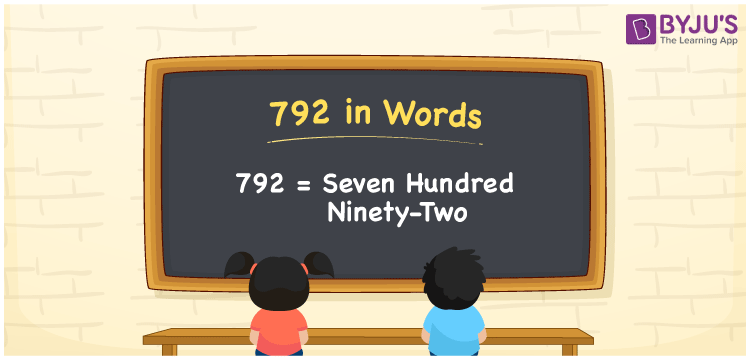# 792 in Words

We can write 792 in words as Seven hundred ninety-two. If you bought a box of candies at Rs. 792, then you can say, “I bought a box of candies at Seven hundred ninety-two rupees”. We can write the word form of the number 792 using a simple place value chart. Here, in this article, you will learn how to write 792 in words using different techniques.

 792 in words Seven hundred ninety-two Seven hundred ninety-two in Numbers 792

## 792 in English Words

We generally use the English alphabet to express numbers in words. So, we can spell 792 in English words as “Seven hundred ninety-two”.## How to Write 792 in Words?

As we can see, 792 is a three-digit number so, let’s create a three-column place value chart such that we can define place values for all digits of 792. This can be done as follows.

 Hundreds Tens Ones 7 9 2

Here, ones = 2, tens = 9, hundreds = 7

These digits can be expanded based on their place values as.

7 × Hundred + 9 × Ten + 2 × One

= 7 × 100 + 9 × 10 + 2 × 1

= 700 + 90 + 2

= Seven hundred + Ninety + Two

= Seven hundred ninety-two

Hence, 792 in words = Seven hundred ninety-two

We know that 792 is a natural number that precedes 793 and succeeds 791.

792 in words – Seven hundred ninety-two

Is 792 an even number? – Yes

Is 792 an odd number? – No

Is 792 a prime number? – No

Is 792 a composite number? – Yes

Is 792 a perfect square number? – No

Is 792 a perfect cube number? – No

## Frequently Asked Questions on 792 in Words

Q1

### How do you spell 792?

You can spell the number 792 using English words as Seven hundred ninety-two.
Q2

### Write 792.500 in words.

We can write the decimal number 792.500 in words as “Seven hundred ninety-two point five zero zero”.
Q3

### How to write Rs. 792 in words on a cheque?

On a cheque, Rs. 792 can be written in words as “Seven hundred ninety-two rupees only”.